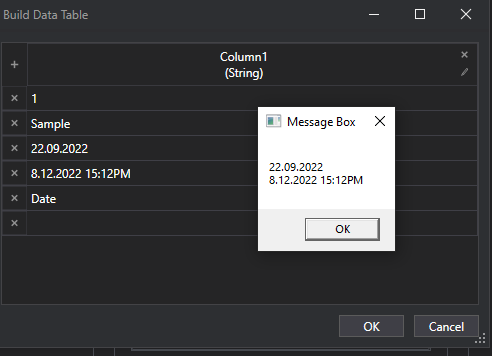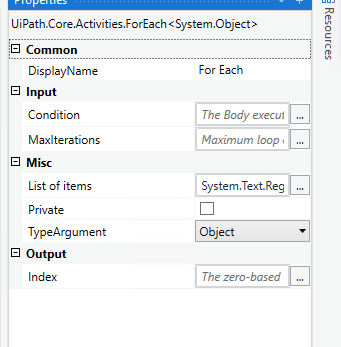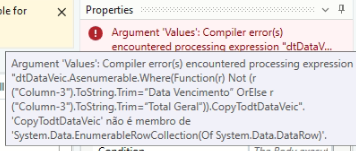# How to extract data in a specific column? Data that falls between two words

How to extract data from a column that is between two lines as in the Image example?
I need to extract to an Array date information that is between the “Data de Vencimento” and “Total Geral”.

You can try this expression to get as string

``````String.join(",",dt.DefaultView.ToTable(true, "Your Column Name").AsEnumerable().Select(Function (a) a(0).ToString).ToArray())
``````

To get as array

``````dt.DefaultView.ToTable(true, "Your Column Name").AsEnumerable().Select(Function (a) a(0).ToString).ToArray()
``````

If you want duplicate you can use False Instead of True

If you dont want duplicates use True

Regards
Sudharsan

You can add the If Condition for checking if that current row item can be converted to DateTime or not.
So It will perform the action only on DateTime .

@Marcelo_Melo
To get the dates between you try like this

``````System.Text.RegularExpressions.Regex.Matches(String.join(",",dt.DefaultView.ToTable(true, "Your Column Name").AsEnumerable().Select(Function (a) a(0).ToString).ToArray()),"(?s)Data de Vencimento,(.*?),Total Geral")
``````

This will give you all the dates that are available in that specific colum which are between “Data de Vencimento” and “Total Geral”.

To use each match you can use for each and can use all the matches like this

If you need only one match you can try with this

``````System.Text.RegularExpressions.Regex.Match(String.join(",",dt.DefaultView.ToTable(true, "Your Column Name").AsEnumerable().Select(Function (a) a(0).ToString).ToArray()),"(?s)Data de Vencimento,(.*?),Total Geral").Tostring
``````

``````System.Text.RegularExpressions.Regex.Match(StringInput,"(?s)Sample(.*?)Date").Groups(1).ToString.Trim
``````

Check out the XAML file

GetValueInBetweenDt.xaml (7.2 KB)

OutputRegards
Gokul

1 Like

Check the updated one

``````
System.Text.RegularExpressions.Regex.Matches(String.join(",",dt.DefaultView.ToTable(true, "Your Column Name").AsEnumerable().Select(Function (a) a(0).ToString).ToArray()),"(?<=Data de Vencimento,).*(?=,Total Geral")
``````

Regards
Sudharsan

1 Like

use this in the for each @Marcelo_Melo

1 Like

Hi!
I got it with the suggestion System.Text.RegularExpressions.Regex.Matches(String.join(“,”,dtDataVeic.DefaultView.ToTable(False, “Column-3”).AsEnumerable().Select(Function (a) a(0 ).ToString).ToArray()),“(?s)Expiration Date,(.*?)Grand Total”)
the return follows below:Is there a way to not extract the lines with the words “Data Vencimento” and “Total Geral”?
How to use the assign activity to be able to use only the lines extracted individually?

My intention is to find only the rows extracted individually to select a CheckBox that exists for each Row

DT.Asenumerable.Where(Function(r) Not (r(ColumnName).ToString.Trim=“Data Vencimento” OrElse r(ColumnName).ToString.Trim=“Total Geral”)).Copytodatatable

Have you tried with my workflow @Marcelo_Melo , Its working ah?

Have you tried this one @Marcelo_Melo ?

Yes, I tried and the error is as follows:

Hi.
Shows this errorHave you checked this XAML file @Marcelo_Melo

It will as you expected

Use “)” at the end of the pattern

Try with this

``````System.Text.RegularExpressions.Regex.Matches(String.join(",",dt.DefaultView.ToTable(true, "Your Column Name").AsEnumerable().Select(Function (a) a(0).ToString).ToArray()),"(?<=Data de Vencimento,).*(?=,Total Geral)")
``````

Regards
Sudharsan